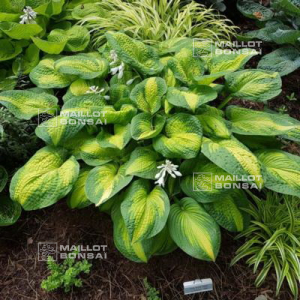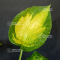##### The Japanese Bonsai specialist
Direct order Contact Help / Services Newsletter# Hosta brother stefan

Hosta brother stefanref. : 11040

12,00

Available quantity : 7Order

###### Description

Coming from a hybridization of Hosta King tut and Mildred seaver.

Dimensions of the leaves : 25/30 cm long x 20/25 cm large.

The leaves are heart shaped and strongly corrugated and full of clear green at the center and darker green on the large sides, measuring 5 to 7 cm.

The main asset of this variety is that the center of the leaves is resistant to burns in spring.

White flowers from the end of June to the middle of July.

Big rhizome delivered in a 1 liter plastic pot.

#leaves 3.6 #hosta 3.5 #brother 2.7 #stefan 2.6 #center 2.6 #hostas 2.6 #green 2.5 #large 2.5 #about 2.5 #from 2.4

###### Technical description
 height 50-70 cm

Formule
(( ROUND((CHAR_LENGTH(b.article_nom)-CHAR_LENGTH(REPLACE(b.article_nom, 'leaves', '')))/LENGTH('leaves')) + ROUND((CHAR_LENGTH(b.article_description)-CHAR_LENGTH(REPLACE(b.article_description, 'leaves', '')))/LENGTH('leaves')) ) * 3.6) + (( ROUND((CHAR_LENGTH(b.article_nom)-CHAR_LENGTH(REPLACE(b.article_nom, 'hosta', '')))/LENGTH('hosta')) + ROUND((CHAR_LENGTH(b.article_description)-CHAR_LENGTH(REPLACE(b.article_description, 'hosta', '')))/LENGTH('hosta')) ) * 3.5) + (( ROUND((CHAR_LENGTH(b.article_nom)-CHAR_LENGTH(REPLACE(b.article_nom, 'brother', '')))/LENGTH('brother')) + ROUND((CHAR_LENGTH(b.article_description)-CHAR_LENGTH(REPLACE(b.article_description, 'brother', '')))/LENGTH('brother')) ) * 2.7) + (( ROUND((CHAR_LENGTH(b.article_nom)-CHAR_LENGTH(REPLACE(b.article_nom, 'stefan', '')))/LENGTH('stefan')) + ROUND((CHAR_LENGTH(b.article_description)-CHAR_LENGTH(REPLACE(b.article_description, 'stefan', '')))/LENGTH('stefan')) ) * 2.6) + (( ROUND((CHAR_LENGTH(b.article_nom)-CHAR_LENGTH(REPLACE(b.article_nom, 'center', '')))/LENGTH('center')) + ROUND((CHAR_LENGTH(b.article_description)-CHAR_LENGTH(REPLACE(b.article_description, 'center', '')))/LENGTH('center')) ) * 2.6) + (( ROUND((CHAR_LENGTH(b.article_nom)-CHAR_LENGTH(REPLACE(b.article_nom, 'about', '')))/LENGTH('about')) + ROUND((CHAR_LENGTH(b.article_description)-CHAR_LENGTH(REPLACE(b.article_description, 'about', '')))/LENGTH('about')) ) * 2.5) + (( ROUND((CHAR_LENGTH(b.article_nom)-CHAR_LENGTH(REPLACE(b.article_nom, 'green', '')))/LENGTH('green')) + ROUND((CHAR_LENGTH(b.article_description)-CHAR_LENGTH(REPLACE(b.article_description, 'green', '')))/LENGTH('green')) ) * 2.5) + (( ROUND((CHAR_LENGTH(b.article_nom)-CHAR_LENGTH(REPLACE(b.article_nom, 'large', '')))/LENGTH('large')) + ROUND((CHAR_LENGTH(b.article_description)-CHAR_LENGTH(REPLACE(b.article_description, 'large', '')))/LENGTH('large')) ) * 2.5) + (( ROUND((CHAR_LENGTH(b.article_nom)-CHAR_LENGTH(REPLACE(b.article_nom, 'from', '')))/LENGTH('from')) + ROUND((CHAR_LENGTH(b.article_description)-CHAR_LENGTH(REPLACE(b.article_description, 'from', '')))/LENGTH('from')) ) * 2.4) + (( ROUND((CHAR_LENGTH(b.article_nom)-CHAR_LENGTH(REPLACE(b.article_nom, 'hybridization', '')))/LENGTH('hybridization')) + ROUND((CHAR_LENGTH(b.article_description)-CHAR_LENGTH(REPLACE(b.article_description, 'hybridization', '')))/LENGTH('hybridization')) ) * 2.3)

## Secure payment## Delivery

Our logistic partners :04 74 55 23 48
Pépinière MAILLOT-BONSAÏ
Le Bois Frazy
01990 RELEVANT - FRANCE
on appointment